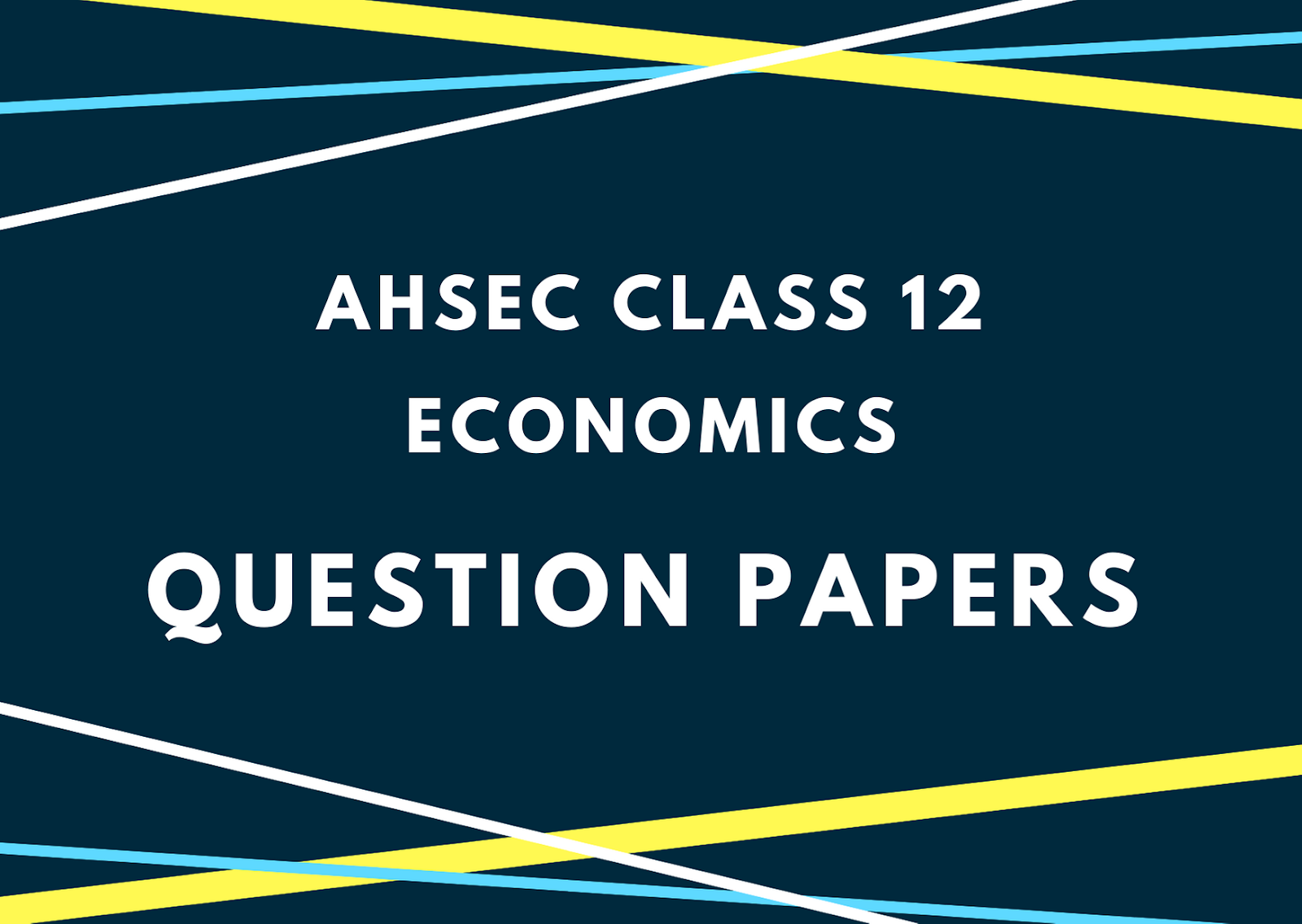# AHSEC Class 12 Economics Question Paper 2017## AHSEC Class 12 Economics Question Papers 2017ACCOUNTANCYFull Marks: 100Pass Marks: 30Time: Three HoursFull Marks: 100 Time: 3 hour
1. (a) Define Service. 1
(b) Fill in the blank:
The allocation of ____ resources and distribution of the final goods and services are the central problems of any economy. 1
(c) What is a demand function? 1
(d) What happens to total product when marginal product is zero? 1
(e) What does a vertical supply curve imply? 1
(f) Why is average total cost (ATC) greater than average variable cost (AVC)? 1
2. State the meaning of microeconomics and macroeconomics.
3. Why does a budget line slope downward?
4. If the total utilities of 4 and 5 units of a commodity for a consume are 56 and 60 respectively, calculate the marginal utility of 4 units of it for him.
5. State any two exceptions of the law of demand. 2
6. Define the term ‘long-run’ as used in production. 2
7. State any two assumptions of the law of variable proportions. 2
8. Distinguish between supply and stock. 4
Or
Explain briefly any four factors affecting supply of a commodity. 4
9. Explain the law of supply with the help of a supply schedule. 4
10. State the relationship between average cost (AC) and marginal cost (MC) using diagram. 4
11. State the distinction between explicit cost and implicit cost. Give one example of each of them. 2+2=4
12. Write down three characteristics of monopolistic competition. State whether the output produced by a firm under such a market is higher/lower than or equal to that of a firm under perfect competition. 3+1=4
Or
“Monopoly firm is a price market” – Explain. 4
13. Explain the two basic conditions of consumer’s equilibrium assuming that the consumers only two goods.
Or
Explain the concepts of change in quantity demanded and change in demand using suitable diagrams.
14. The demand and supply functions of a firm under perfectly competitive market are given below –Find (i) The equilibrium price and output.
(ii), what will be the changes in equilibrium Price and Output? 3+3=6
Or
The total cost (TC) and prices (P) at different units of output of a monopolist are given below:
1. Find out the total revenue (TR), marginal revenue (MR) and marginal cost (MC) schedules.
2. Find out the equilibrium quantity of output. 1+2+2+1=6
 Q TC P 1 2 3 4 5 6 7 8 20 35 45 51 58 66 76 88 15 14 13 12 11 10 9 8

### Also Read: AHSEC Class 12 Economics Question Paper15. (a) What is the alternative name of macroeconomics? 1
(b) What is the significance of the 450 line in Keynesian income determination model? 1
(c) What is the value of MPC and MPS is zero? 1
(d) What is foreign exchange rate? 1
(e) What is meant by appreciation of the currency of a country? 1
(f) What is invisible trade? 1
16. Give any two examples of macroeconomics variable. 2
17. State the concept of depreciation in the context of national income accounting. 2
18. If the marginal propensity to save (s) of an economy is 0.3, find out the value of the income multiplier. 2
19. State any two measures of fiscal policy to correct, the problem of excess demand in an economy. 2
20. Give the concept of full employment equilibrium. 2
21. What is deficit financing? 2
22. What are the four factors of production? Write down the name of the remuneration to each of them. 2+2
23. Explain any four causes of disequilibrium in BOP. 4
Or
Distinguish between factor income and transfer income. 4
24. It is planned to increase national income by Rs. 1,000 crore in an economy. How much increase in investment is required to achieve this goal if MPC = 0.6? 4
25. What is the meaning of government budget? Distinguish between revenue receipts and capital receipts. 1+3
26. Point out two merits and two demerits of indirect tax. 2+2=4
27. Explain the income method of calculating GDP. 6
Or
Explain the relationship between investment multiplier and MPC. 6
28. Briefly explain any four functions of a commercial bank. 6
Or
Describe the speculative demand for money. 6